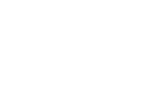# Solutions

Get detailed explanations to advanced GMAT questions.

### QuestionIn the triangle above, is x > 90?

I. a^2 + b^2 <15

II. c > 4

Option A:

Statement (1) ALONE is sufficient, but statement (2) alone is not sufficient to answer the question asked.

Option B:

Statement (2) ALONE is sufficient, but statement (1) alone is not sufficient to answer the question asked.

Option C:

BOTH statements (1) and (2) TOGETHER are sufficient to answer the question asked, but NEITHER statement ALONE is sufficient to answer the question asked.

Option D:

Option E:

Statements (1) and (2) TOGETHER are NOT sufficient to answer the question asked, and additional data specific to the problem are needed.

Medium

### Solution

Option C is the correct answer.

### Option Analysis

Each statement alone is clearly insufficient. When taken together:

If angle x were 90 degrees than we would have a^2+b^2=4^2, since a^2+b^2<15<16 then angle x must be greater than 90 degrees (c^2 is greater than a^2+b^2 then the angel opposite c must be greater than 90).